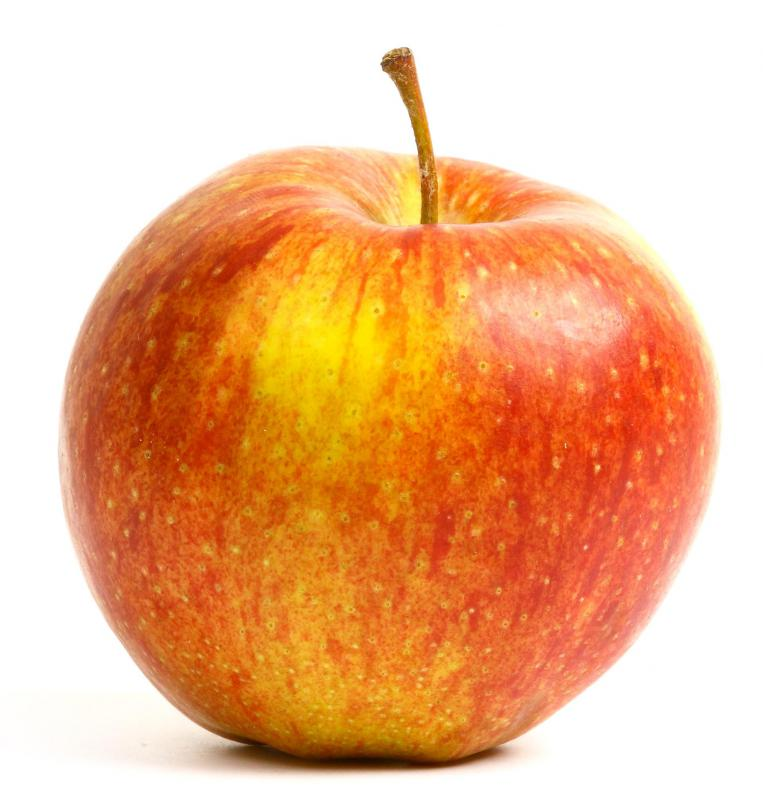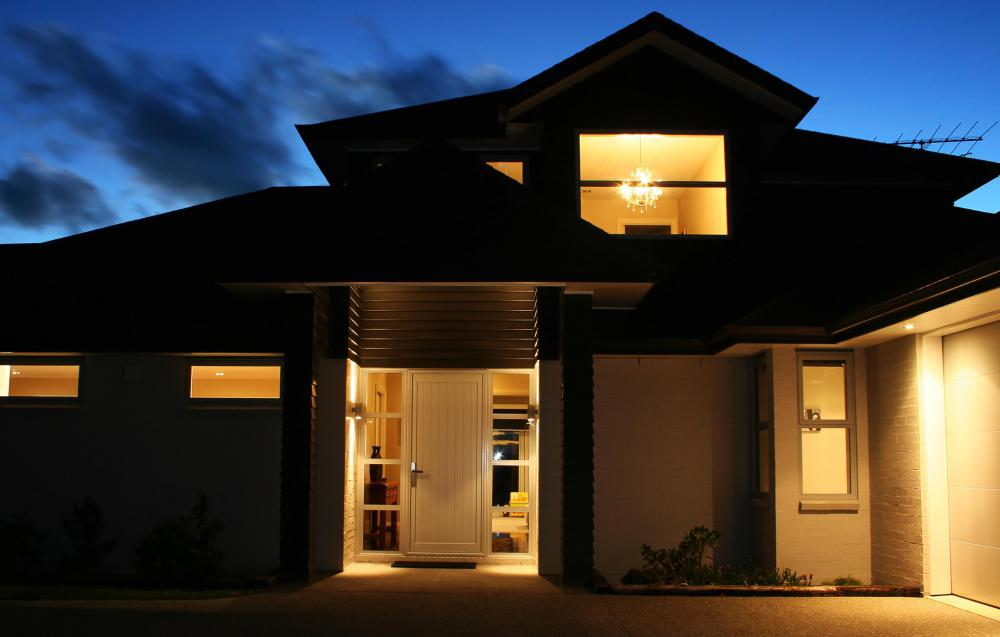# What is a Joule?

Niki Foster
Niki Foster

The joule (J) is the unit of measure for energy used in the International System of Units (SI), and the one accepted in scientific circles. The unit is used in all equations and calculations involving energy. Following SI conventions, joules are abbreviated with a capital J because the term is derived from a proper name, though if spelled out, it is not capitalized. One J is defined as the amount of energy expended by a force of 1 newton moving an object 1 meter in the same direction as the force. A newton is the force required to accelerate a mass of 1 kilogram (2.2 pounds) at a rate of 1 meter (3.3 feet) per second per second; therefore, the acceleration is such that the speed of the mass increases by 1 meter per second with each second that passes.

#### Definition

The above definition of a joule may seem complicated, but the advantage about SI units is that they enable all the many things that scientists might want to measure to be quantified in terms of a small number of base units. It also means that, by sticking with these units, all scientists are speaking the same language. The joule is said to be a derived unit, in that it is obtained from the base units kilograms, meters, and seconds.The amount of energy required to lift an apple 1 meter (3.3 feet) is roughly equivalent to 1 Joule.

It can be regarded as a newton-meter — a force of 1 newton exerted over a distance of 1 meter (3.3 feet). Since the newton is itself a derived unit, a joule can be further broken down as meters squared times kilograms times meters per second per second:

J = m2kgs-2

Although a joule is 1 newton-meter, energy is not normally referred to using the latter unit, to avoid confusion with the unit of measure for torque, or moment of force, which has to do with rotational motion.

#### Force, Work, Energy and PowerIndividuals pay per kilowatt hour for the electricity they use in their homes.

There is often some confusion between these terms, and the units used to measure them. A force is an influence that causes a change in the motion of an object; in other words, acceleration. Work is the exertion of force to produce movement, while energy is the potential to do work, and is expended when work is done. For example, pushing an object in a certain direction involves applying a force, which does work, and energy must be used up. Work and energy can both be measured in joules.

Power is the rate at which energy is used up and is measured in watts. Using up 1 J of energy for one second uses 1 watt of power, so 1 watt is 1 joule per second. A 100-watt light bulb uses 100 J of energy per second. Household electricity consumption is measured in kilowatt-hours: a kilowatt is 1,000 watts and 1 kilowatt hour is 3,600,000 J.

#### Examples

To get an idea of how much energy a joule actually is, it helps to look at some everyday examples. A joule is the amount of energy needed to raise the temperature of 1 gram of cool, dry air by 1.8°F (1.0°C). An average person sitting quietly produces 100 J of heat every second. The amount of energy required to lift a small apple 1 meter (3.3 feet) against the Earth's gravity is roughly equivalent to 1 J.

#### History

The unit is named after the English physicist James Prescott Joule, whose work led to the theory of conservation of energy, and the first law of thermodynamics, in the 19th century. Through his experiments, he was able to determine that energy changes from one form to another when work is done, but cannot be created from nothing or destroyed. For example, when electricity is used to turn a motor, part of the electrical energy is turned into motion, and the rest is converted into heat. He was able to show that the motion plus the heat were equivalent to the original amount of electrical energy.Niki Foster

In addition to her role as a InfoBloom editor, Niki enjoys educating herself about interesting and unusual topics in order to get ideas for her own articles. She is a graduate of UCLA, where she majored in Linguistics and Anthropology.Niki Foster

In addition to her role as a InfoBloom editor, Niki enjoys educating herself about interesting and unusual topics in order to get ideas for her own articles. She is a graduate of UCLA, where she majored in Linguistics and Anthropology.

## You might also Likeanon989287

joule = N*m or kg*m/s^2*m

anon951942

What are the different types of energy?

anon947611

@David09 and @SkyWhisperer: Calories are a measurement of heat, which is a form of energy.

anon352955

Is a Joule equal to a Newton*(m)?

amdewster

How many joules are required to cause air (and water) to break down into plasma? Anybody?

anon301292

what is kinetic energy?

anon301277

Watts are never equivalent to joules, because watt is a unit for *power* and joule is a unit for *energy*. But why are newton-metres not equivalent to joules?

anon274569

In daily use terms, one 99999 watt lamp, how many joules is it equivalent to? Is it 360 joules?

nony

@SkyWhisperer - Now you know what all those joule units are every time that you go looking for a power surge protector. They usually tell you that the surge strip will protect you up to 15,000 joules or something like that.

Sometimes they will give you a comparison by telling it’s comparable to a direct hit from a lightning strike. When I buy surge protectors I always make it a point to go for the highest joule protection.

There’s no reason to skimp really. My computer equipment is worth well over \$1,000. I can afford to buy the best quality surge protector I can find for the money.

SkyWhisperer

@David09 - I believe that there's a formulaic correlation. Since according to the article one of the elements in the formula is mass (grams), then your weight would have some impact.

If you had significant mass then you would expend more joules I would think. I don’t know what the caloric impact of the joule you expend would be. But I don’t think that there is a direct correlation between those two numbers as you suggest.

David09

Well this is an interesting explanation of joule conversions. I can now finally quantify the impact of my solitude. As a quiet person, I emit 100 joules of energy every second!

I am not sure if that’s a big or a high number, but I’ll bet that it’s on the low side since you don’t expend a lot of energy to be quiet. It reminds me of the figure that I once read about how many calories you burn just by sitting still.

It’s something like 10 calories or more per hour. Since the amount of joules expended is also a factor of 10 I wonder if there is a direct correlation between burning up calories and the amount of joules you spend?

anon124228

I needed to have my heart rhythm reset. The doctors used 50 joules of electricity. How much was that in laymen's terms?

anon108381

@-anon88878: That is not correct. A Joule is a measure of energy. A watt is a measure of power. A watthour (Wh) is 3,600J not a watt.

anon88878

thus a watt is 3,600J so 100 watts is 360,000J

anon82822

in daily use terms. one 100 watt lamp, how many joules is it equivalent to? is it 360 joules?